## How to Draw Perpendicular and Parallel Lines?

Practice Unlimited Questions

#### 1. What are perpendicular lines?

Two lines meeting or crossing each other at 90° are called perpendicular lines.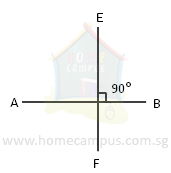In the figure above, the lines AB and EF cross each other at a right angle (90°).

AB and EF are perpendicular to each other. We write it as AB EF.

#### 2. What are parallel lines?

Two or more lines that never meet are called parallel lines.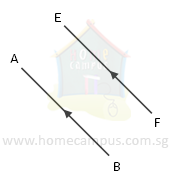In the figure above, the lines AB and EF are parallel lines.

We write it as AB // EF. We show two or more parallel lines using arrowheads.

#### 3. How to draw a perpendicular line?

Use a set square to draw a line that is perpendicular to the line XY and passes through the point P.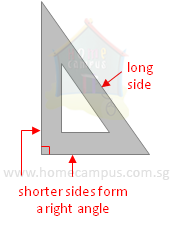Step 1:
Place one short side of the set square on the line XY.Step 2:
Move the set square along XY so the other short side touches Point P.Step 3:
Use the edge of the set square to draw a line through Point P as shown below.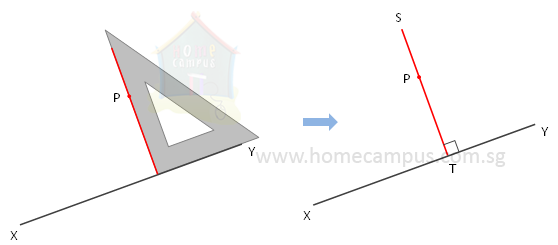In the figure above, ST XY.

#### 4. How to draw a parallel line?

Use a set square to draw a line that is parallel to the line XY and passes through the point P.Step 1:
Place one short side of the set square on the line XY. Then place a ruler on the other short side.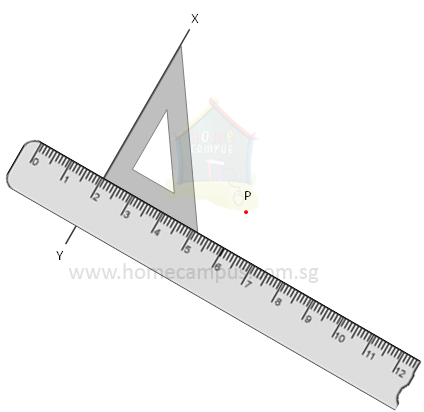Step 2:
Slide the set square along the ruler until the side of the set square that was placed against XY touches Point Q.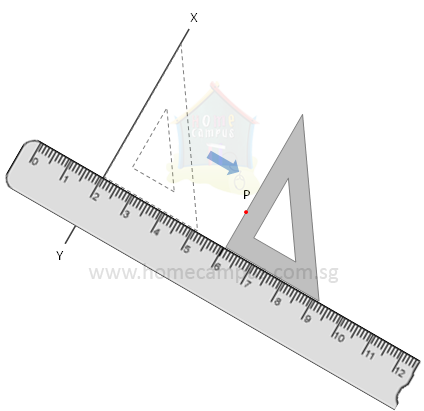Step 3:
Use the edge of the set square to draw a line through Point P as shown below.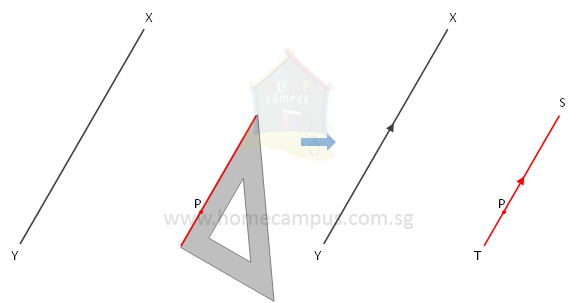In the figure above, ST // XY.

#### 5. Horizontal and Vertical Lines

The figure below shows a rectangular frame hung on a wall.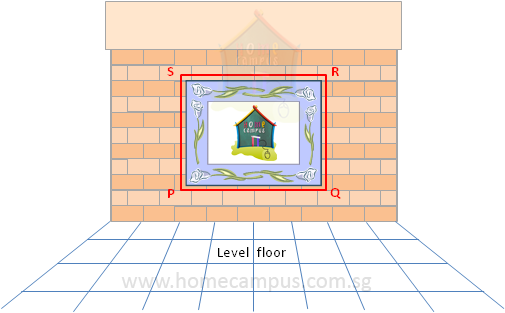The lines PQ and SR are horizontal lines. They are parallel to the level floor. All horizontal lines are parallel to each other.

The lines SP and RQ are vertical lines. They are perpendicular to the level floor. All vertical lines are parallel to each other.

A horizontal line and a vertical line are perpendicular to each other.